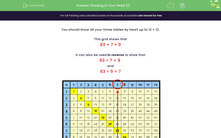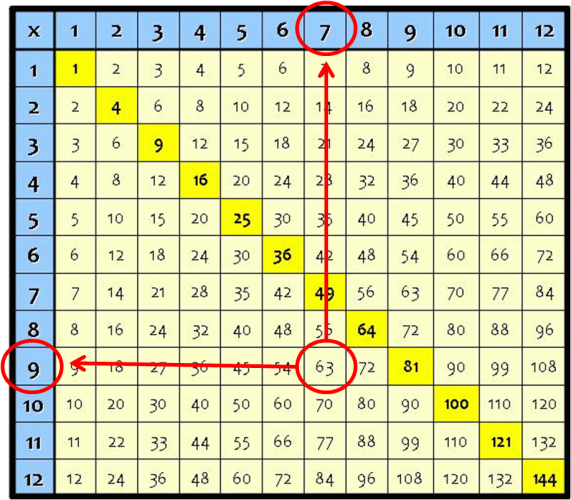# Use Knowledge of Times Tables for Division

In this worksheet, students will use their knowledge of their times tables to divide the given numbers mentally.Key stage:  KS 2

Curriculum topic:   Number: Multiplication and Division

Curriculum subtopic:   Multiply/Divide Mentally

Difficulty level:#### Worksheet Overview

You should know all your times tables by heart up to 12 × 12.

The grid below shows that

63 = 7 × 9

It can also be used in reverse to show that

63 ÷ 7 = 9

and

63 ÷ 9 = 7Can you use it to work out multiplication and division facts for 7 x 8?

7 x 8 = 56

8 x 7 = 56

56 ÷ 8 = 7

56 ÷ 7 = 8

The following questions will test your knowledge of your times tables to see if you can solve division problems in your head. Let's see how you get on.

### What is EdPlace?

We're your National Curriculum aligned online education content provider helping each child succeed in English, maths and science from year 1 to GCSE. With an EdPlace account you’ll be able to track and measure progress, helping each child achieve their best. We build confidence and attainment by personalising each child’s learning at a level that suits them.

Get started